# GSEB Solutions Class 8 Maths Chapter 6 Square and Square Roots InText Questions

Gujarat Board GSEB Textbook Solutions Class 8 Maths Chapter 6 Square and Square Roots InText Questions and Answers.

## Gujarat Board Textbook Solutions Class 8 Maths Chapter 6 Square and Square Roots InText Questions

Try These (Page 90)

Question 1.
Find the perfect square numbers between

1. 30 and 40 and
2. 50 and 60.

Solution:
1. Since,
1 × 1 = 1
2 × 2 = 4
3 × 3 = 9
4 × 4 = 16
5 × 5 = 25
6 × 6 = 36
7 × 7 = 49
Thus, 36 is a perfect square number between 30 and 40.

2. Since, 7 × 7 = 49 and 8 × 8 = 64. It mean there is no perfect number between 49 and 64; and thus there is not perfect number between 50 and 60.

Try These (Page 90)

Question 1.
Can we say whether the following numbers are perfect squares? How do we know?

1. 1057
2. 23453
3. 7928
4. 222222
5. 1069
6. 2061

Write five numbers which you can decide by looking at their ones digit that they are not square numbers?
Solution:
1. 1057
∵ The ending digit is 7 (which is not one of 0, 1, 4, 5, 6 or 9)
∴ 1057 cannot be a square number.

2. 23453
∵ The ending digit is 3 (which is not one of 0, 1, 4, 5, 6 and 9).
∴ 23453 cannot be a square number.

3. 7928
∵ The ending digit is 8 (which is not one of 0, 1, 4, 5, 6 and 9).
∴ 7928 cannot be a square number.

4. 222222
∵ The ending digit is 2 (which is not one of 0, 1, 4, 5, 6 or 9).
∴ 222222 cannot be a square number.

5. 1069
∵ The ending digit is 9.
∴ It may or may not be a square number. Also,
30 × 30 = 900
31 × 31 = 961
32 × 32 = 1024
33 × 33 = 1089
i.e., No natural number between 1024 and 1089 which is a square number.
1069 cannot be a square number.

6. 2061
∵ The ending digit is 1
∴ It may or may not be a square number.
45 × 45 = 2025
and 46 × 46 = 2116
i.e., No natural number between 2025 and
2116 which is a square number.
2061 is not a square number.
We can write many numbers which do not end with 0, 1, 4, 5, 6 or 9. (i.e., which are not square numbers). Five such numbers can be:
1234, 4312, 5678, 87543, 1002007Question 2.
Write five numbers which you cannot decide just by looking at their units digit (or ones place) whether they are square numbers or not?
Solution:
Property 1.
Any natural number ending in 0, 1, 4, 5, 6 or 9 can be or cannot be a square- number. Five such numbers are:
56790, 3671, 2454, 76555, 69209

Property 2.
If a number has 1 or 9 in the units place, then its square ends in 1.

For example:
(1)2 = 1,
(9)2 = 81,
(11)2 = 121,
(19)2 = 361,
(21)2 = 441

Try These (Page 91)

Question 1.
Which of 1232, 772, 822, 1612, 1092 would end with digit 1?
Solution:
The squares of those numbers end in 1 which end in either 1 or 9.
∴ The squares of 161 and 109 would end in 1.

Property 3.
When a square number ends in 6, then the number whose square it is, will have 4 or 6 in its unit place.

Try These (Page 91)

Question 1.
Which of the following numbers would have digit 6 at unit place?

1. 192
2. 242
3. 262
4. 362
5. 342

Solution:
1. 192: Unit place digit = 9
∴ 192 would not have unit digit as 6.

2. 242: Unit place digit = 4
∴ 242 would have unit digit as 6.

3. 262: Unit place digit = 6
∴ 262 would have 6 as unit place.

4. 362: Unit place digit = 6
∴ 362 would end in 6.

5. 342: Since, the unit place digit is 4
∴ 342 would have unit place digit as 6.

Try These (Page 92)

Question 1.
What will be the ‘‘ones digit” in the square of the following numbers?

1. 1234
2. 26387
3. 52698
4. 99880
5. 21222
6. 9106

Solution:
1.
∵ Ending digit = 4 and 42 = 16
∴ (1234)2 will have 6 as the ones digit.

2.
∵ Ending digit is 7 and 72 = 49
∴ (26387)2 will have 9 as the ones digit.

3.
∵ Ending digit is 8, and 82 = 64
∴ (52698)2 will end in 4.

4.
∵ Ending digit is 0.
∴ (99880)2 will end in 0.

5.
∵ 22 = 4
∴ Ending digit of (21222)2 is 4.

6.
∵ 62 = 36
∴ Ending digit of (9106)2 is 6.

Property 4.
A square number can only have even number of zeros at the end.

Property 5.
The squares of odd numbers are odd and the squares of even numbers are even.

Try These (Page 92)

Question 1.
The square of which of the following numbers would be an odd number/an even number? Why?

1. 727
2. 158
3. 269
4. 1980

Solution:
1. 727
Since 727 is an odd number.
∴ Its square is also an odd number.

2. 158
Since 158 is an even number.
∴ Its square is also an even number.

3. 269
Since 269 is an odd number.
∴ Its square is also an odd number.

4. 1980
Since 1980 is an even number.
∴ Its square is also an even number.Question 2.
What will be the number of zeros in the square of the following numbers?

1. 60
2. 400

Solution:
1. In 60, number of zero is 1 .
∴ Its square will have 2 zeros.

2. ∵ There are 2 zeros in 400.
∴ Its square will have 4 zeros.

Property 6.
The difference between the squares of two consecutive natural numbers is equal to the sum of the two numbers.

Property 7.
There are 2n non – perfect square numbers between the squares of the numbers n and n + 1.

Try These (Page 94)

Question 1.
How many natural numbers lie between 92 and 102? Between 112 and 122?
Solution:
(a) Between 92 and 102
Here, n = 9 and n + 1 = 10
∴ Natural numbers between 92 and 102 are (2 × n) or 2 × 9, i.e. 18.

(b) Between 112 and 122
Here, n = 11 and n + 1 = 12
Natural numbers between 112 and 122 are (2 × n) or 2 × 11, i.e. 22.Question 2.
How many non-square numbers lie between the following pairs of numbers:

1. 1002 and 1012
2. 902 and 912
3. 10002 and 10012

Solution:
1. Between 1002 and 1012
Here, n = 100
∴ n × 2
= 100 × 2
= 200
∴ 200 non-square numbers lie between 1002 and 1012.

2. Between 902 and 912
Here, n = 90
∴ 2 × n = 2 × 90 or 180
∴ 180 non-square numbers lie between 90 and 91.

3. Between 10002 and 10012
Here, n = 1000
∴ 2 × n = 2 × 1000 or 2000
∴ 2000 non-square numbers lie between 10002 and 10012.

Property 8.
The sum of first n odd natural numbers is n2
or
If there is a square number, it has to be the sum of the successive odd numbers starting from 1.

Try These (Page 94)

Question 1.
Find whether each of the following numbers is a perfect square or not?

1. 121
2. 55
3. 81
4. 49
5. 69

Solution:
REMEMBER
If a natural number cannot be expressed as a sum of successive odd natural numbers starting from 1, then it is not a perfect square.

1. 121
Since, 121 – 1 = 120
85 – 13 = 72
120 – 3 = 117
72 – 15 = 57
117 – 5 = 112
57 – 17 = 40
112 – 7 = 105
40 – 19 = 21
105 – 9 = 96
21 – 21 = 0
96 – 11 = 85
i.e., 121 = 1 + 3 + 5 + 7 + 9 + 11 + 13 + 15 + 17 + 19 + 21. Thus, 121 is a perfect square.

2. 55
Since, 55 – 1 = 54
54 – 3 = 51
51 – 5 = 46
46 – 7 = 39
Since, 55 cannot be expressed as the sum of successive odd numbers starting from 1.
∴ 55 is not a perfect square.

3. 81
Since, 81 – 1 = 80
56 – 11 = 45
80 – 3 = 77
77 – 5 = 72
72 – 7 = 65
65 – 9 = 56
∴ 81 = 1 + 3 + 5 + 7 + 9 + 11 + 13 + 15 + 17.
Thus, 81 is a perfect square.

4. 49
Since,
49 – 1 = 48
33 – 9 = 24
48 – 3 = 45
24 – 11 = 13
45 – 5 = 40
13 – 13 = 0
40 – 7 = 33
∴ 49 = 1 + 3 + 5 + 7 + 9+ 11 + 13
Thus, 49 is a perfect square.

5. 69
Since, 69 – 1 = 68
44 – 11 = 33
68 – 3 = 65
33 – 13 = 20
65 – 5 = 60
20 – 15 = 5
60 – 7 = 53
5 – 17 = -12
53 – 9 = 44
∴ 69 cannot be expressed as the sum of consecutive odd numbers starting from 1.
Thus, 69 is not a perfect square.

Property 9.
The square of an odd number can be expressed as the sum of two consecutive natural numbers.

Try These (Page 95)

Question 1.
Express the following as the sum of two consecutive integers.

1. 212
2. 132
3. 112
4. 192

Solution:
1. n = 21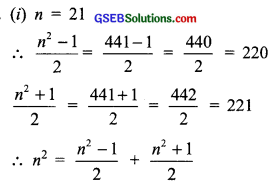or 212 = 220 + 221 = 441

2. n = 13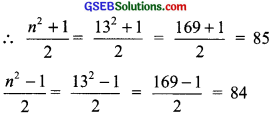∴ 132 = 85 + 84 = 169
Similarly,

3. 112 = 60 + 61 = 121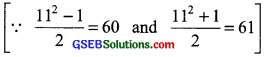4. 192 = 180 + 181 = 361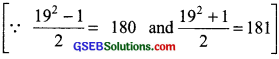Question 2.
Do you think the reverse is also true, i.e., is the sum of any two consecutive positive integers is perfect square of a number? Give example to support your answer?
Solution:
No, it is not always true.
For example:

• 5 + 6 = 11, 11 is not a perfect square.
• 21 + 22 = 43, 43 is not a perfect square.

Property 10.
The difference between the squares of two consecutive natural numbers is equal to the sum of the two numbers?
Examples:
92 – 82 = 81 – 64 = 17 = 9 + 8
102 – 92 = 100 – 81 = 19 = 10 + 9
152 – 142 = 225 – 196 = 29 = 15 + 14
1012 – 1002 = 10201 – 10000 = 201 = 101 + 100

Property 11.
If (n + 1) and (n – 1) are two consecutive even or odd natural numbers, then (n + 1) × (n – 1) = n2 – 1.
For example,
10 × 12 = (11 – 1) × (11 + 1) = 112 – 1
11 × 13 = (12 – 1) × (12 + 1) = 122 – 1
25 × 27 = (26 – 1) × (26 + 1) = 262 – 1

Try These (Page 95)

Question 1.
Write the square, making use of the above pattern?

1. 1111112
2. 111111112

Solution:
Using above pattern we can write,

1. (111111)2 = 12345654321
2. (11111111)2 = 1234567654321Question 2.
Can you find the square of the following numbers using the above pattern?

1. 66666672
2. 666666672

Solution:
Using the above pattern, we can have,

1. 66666672 = 44444448888889
2. 666666672 = 4444444488888889

Try These (Page 97)

Question 1.
Find the square of the following numbers containing 5 in unit place?

1. 15
2. 95
3. 105
4. 205

Solution:
1. (15)2 = 1 × (1 + 1) × 100 + 25
= 1 × 2 × 100 + 25
= 200 + 25 = 225

2. (95)2 = 9(9 + 1) × 100 + 25
= 9 × 10 × 100 + 25
= 9000 + 25 = 9025

3. (105)2 = 10 × (10 + 1) × 100 + 25
= 10 × 11 × 100 + 25
= 11000 + 25 = 11025

4. (205)2 = 20 × (20 + 1) × 100 = 25
= 20 × 21 × 100 + 25 = 42000 + 25 = 42025

Try These (Page 99)

Question 1.

1. 112 = 121. What is the square root of 121?
2. 142 = 196. What is the square root of 196?

Solution:

1. The square root of 121 is 11.
2. The square root of 196 is 14.

Try These (Page 99)

Question 1.
(-1)2 = 1. Is -1, a square root of 1?
(-2)2 = 4. Is -2, a square root of 4?
(-9)2 = 81. Is -9, a square root of 81?
Solution:
1. Since (-1) × (-1) = 1
i.e., (-1)2 = 1
Square root of 1 can also be -1.

Similarly,
2. Yes, (-2) is a square root of 4.
3. Yes, (-9) is a square root of 81.
Since, we have to consider the positive square roots only and the symbol for a positive square root is $$\sqrt{}$$.
∴ $$\sqrt{16}$$ = 4 [and not (-4)]
Similarly, $$\sqrt{25}$$ means, the positive square root of 25, i.e., 5.Try These (Page 100)

Question 1.
By repeated subtraction of odd numbers starting from 1, find whether the following numbers are perfect squares or not? If the number is a perfect square, then find its square root?

1. 121
2. 55
3. 36
4. 49
5. 90

Solution:
1. Subtracting the successive odd numbers from 121, we have
120 – 1 = 120
120 – 3 = 117
117 – 5 = 112
112 – 7 = 105
105 – 9 = 96
96 – 11 = 85
85 – 13 = 72
72 – 15 = 57
57 – 17 = 40
40 – 19 = 21
21 – 21 = 0
∴ $$\sqrt{121}$$ = 11.
[∵ We had to subtract the first 11 odd numbers.]

2. ∵ 55 – 1 = 54
51 – 5 = 46
39 – 9 = 30
19 – 13 = 6
54 – 3 = 51
46 – 7 = 39
30 – 11 = 19
6 – 15 = -9
and we do not reach to 0.
∴ 55 is not a perfect square.

3. ∵ 36 – 1 = 35
35 – 3 = 32
32 – 5 = 27
27 – 7 = 20
20 – 9 = 11
11 – 11 = 0
and we have obtained 0 after subtracting 6 successive odd numbers.
∴ 36 is a perfect square.
Thus, $$\sqrt{36}$$ = 6.

4. We have
49 – 1 = 48
48 – 3 = 45
45 – 5 = 40
40 – 7 = 33
33 – 9 = 24
24 – 11 = 13
13 – 13 = 0

5. We have:
90 – 1 = 89
89 – 3 = 86
86 – 5 = 81
81 – 7 = 74
74 – 9 = 65
65 – 11 = 54
54 – 13 = 41
41 – 15 = 26
26 – 17 = 9
9 – 19 = -10
Since, we can not reach to 0 after subtracting successive odd numbers.
∴ 90 is not a perfect square.Try These (Page 103)

Question 1.
Can we say that if a perfect square is of n-digits, then its square root will have $$\frac{n}{2}$$ digits if n is even or $$\frac{(n+1)}{2}$$ if n is odd?
Solution:
Yes, it is true that: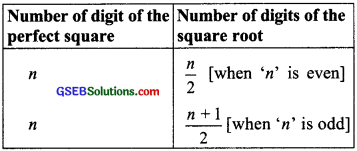Examples:
1. 529 (a perfect number),
n = 3 (odd number)
∴ Number of digits in square root
= $$\frac{n+1}{2}$$ = $$\frac{3+1}{2}$$ = 2
Also, square root of 529 = 23 (2-digits)

2. 1296 (is a perfect square) and n
= 4 (even number)
Number of digits of its square root
= $$\frac{n}{2}$$ = $$\frac{4}{2}$$ = 2
Now $$\sqrt{1296}$$ = 36 (2-digits)

Try These (Page 105)

Question 1.
Without calculating square roots, find the number of digits in the square root of the following numbers?

1. 25600
2. 100000000
3. 36864

Solution:
1. 25600
∵ n = 5 [an odd number]
∴ Its square root will have $$\frac{n+1}{2}$$ digits,
i.e; $$\frac{5+1}{2}$$ = $$\frac{6}{2}$$ = 3 digits

2. 100000000
∵ n = 9 → odd number
∴ Number of digits of its square root
= $$\frac{n+1}{2}$$ = $$\frac{9+1}{2}$$ = $$\frac{10}{2}$$ = 5

3. 36864
∵ n = 5 → odd number
∴ Number of digits in its square root
= $$\frac{n+1}{2}$$ = $$\frac{5+1}{2}$$ = $$\frac{6}{2}$$ = 3Try These (Page 107)

Question 1.
Estimate the value of the following to the nearest whole number?

1. $$\sqrt{80}$$
2. $$\sqrt{1000}$$
3. $$\sqrt{350}$$
4. $$\sqrt{500}$$

Solution:
1. $$\sqrt{80}$$
∵ 102 = 100,
92 = 81,
82 = 64
and 80 is between 64 and 81.
i.e., 64 < 80 < 81
or 82 < 80 < 92
or 8 < $$\sqrt{80}$$ < 9
Thus, $$\sqrt{80}$$ lies between 8 and 9.

2. $$\sqrt{1000}$$
We know that
302 = 900, 312 = 961, 322 = 1024
∴ 1000 lies between 961 and 1024.
i.e., 916 < 1000 < 1024
or 312 < 1000 < 322
or 31 < $$\sqrt{1000}$$ < 32
Thus, $$\sqrt{1000}$$ lies between 31 and 32.

3. $$\sqrt{350}$$
We have 182 = 324,
192 = 361
Since, 350 lies between 324 and 316
or 324 < 350 < 361
or 182 < 350 < 192
or 18 < $$\sqrt{350}$$ < 19
Thus, $$\sqrt{350}$$ lies between 18 and 19.

4. $$\sqrt{500}$$
∵ 222 = 484 and 232 = 529
Since, 500 lies between 484 and 529.
or 484 < 500 < 529
0r 222 < 500 < 232
or 22 < $$\sqrt{500}$$ < 23
∴$$\sqrt{500}$$ lies between 22 and 23.